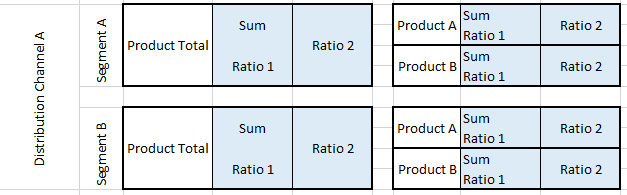cancel
Showing results for
Did you mean:Frequent Visitor

## Table as Filter in CALCULATE

Hello,
first of all: the introduction takes a little longer, because I have to explain why we do what.
We need to create a report at our company that needs to show 3 values each for multiple distribution channels and multiple segments and products. There are 106 visuals in total. Each of these visuals is a multirow (some contain 2 values, some only 1 value). The visuals have different conditional formatting behind them, hence the 2 to 1 split.

Here is a short rough representation of how something like this looks. Each of the blue boxes is 1 visual:This representation repeats for all distribution channels.

The idea was that in each visual individually the filters are set. But I want to avoid setting the filters manually in 106 visuals. My idea was to create a measure for the metrics, which I can reuse:

``Sum = SUM(Table[sum])``
``Ratio 1 = DIVIDE(SUM(Table[sum2]);SUM(Table[sum3]))``
``Ratio 2 = DIVIDE(SUM(Table[sum4]);SUM(Table[sum3]))``

This is the structure of the measure I would create for all combinations:

``````Distribution_Channel_1_Total =
CALCULATE(
[Sum];
Table[distribution_channel] = "A";
Table[segment] = "Segment A";
Table[product] = "Product A"))``````

My question is, is it possible to use a table in Calculation as a filter? A table that contains all necessary attributes and via an ID these attributes are taken into account in the Calculate function.``````Distribution_Channel_1_Total =
CALCULATE(
[Sum];
table_with_values = 1)``````

I hope I was able to explain my request reasonably clearly.

Thank you very much in advance!

Markus

1 ACCEPTED SOLUTIONSuper User

You could try something like

``````Distribution channel 1 total =
VAR FilterTable =
CALCULATETABLE (
SUMMARIZE (
'Filter table',
'Filter table'[distribution channel],
'Filter table'[segment],
'Filter table'[product]
),
'Filter table'[id] = 1
)
VAR Result =
CALCULATE (
[Sum],
TREATAS (
FilterTable,
'Table'[distribution channel],
'Table'[segment],
'Table'[product]
)
)
RETURN
Result
``````
2 REPLIES 2Super User

You could try something like

``````Distribution channel 1 total =
VAR FilterTable =
CALCULATETABLE (
SUMMARIZE (
'Filter table',
'Filter table'[distribution channel],
'Filter table'[segment],
'Filter table'[product]
),
'Filter table'[id] = 1
)
VAR Result =
CALCULATE (
[Sum],
TREATAS (
FilterTable,
'Table'[distribution channel],
'Table'[segment],
'Table'[product]
)
)
RETURN
Result
``````Frequent Visitor

Hi @johnt75 ,

great, thank you very much! This is exactly what I was looking for!Announcements#### Exclusive opportunity for Women!

Join us for a free, hands-on Microsoft workshop led by women trainers for women where you will learn how to build a Dashboard in a Day!#### Power Platform Conference-Power BI and Fabric Sessions

Join us Oct 1 - 6 in Las Vegas for the Microsoft Power Platform Conference.Top Solution Authors
Top Kudoed Authors
Users online (3,662)